# Percentages

Percent means out of 100; the symbol for percentage is %. The percentage of a whole birthday cake is 100%.

100%50%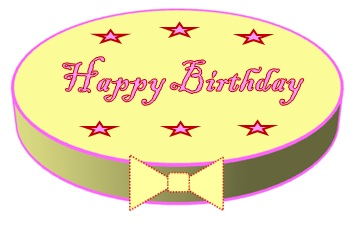Half the cake would
be 50% or 50/100
Remember!
Percent means out
of 100. We can
simplify 50/100 to 1/2.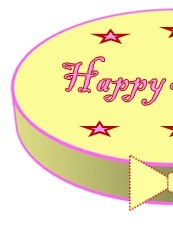100%
 50% 50%

 25% 25% 25% 25%
 12.5% 12.5% 12.5% 12.5% 12.5% 12.5% 12.5% 12.5%

## Percentage of an Item

Q.1 What is 25% of an item that costs ?300?

We know 100% of the item is ?300 so 25% of the item would be 25/100 x ?300.

We are going to look at two different methods to calculate the result.

 Method 1 Step1: Write the percentage as a fraction.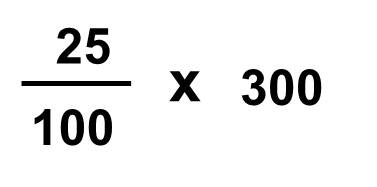Step 2: Cancel out the zeros.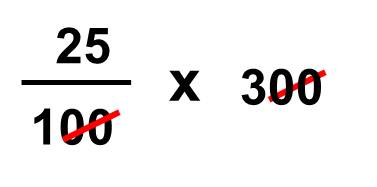Step 3: Multiply 25 by 3.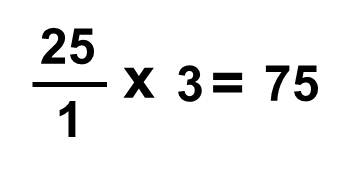Answer = ?75 Method 2 10% of 300 = 30 20% would be 30 x 2 =60 5% would be 30/2= 15 25% would be: (60 + 15 = 75) Answer= ?75

Q.2 The value of an item is worth ?220 within a year it increases by 20%.
What is the new value of the item?

1. 10% of 220 = 22
20% would be 22 x 2 = 44

2. The value has increased by ?44
so the new value will be the original price plus the 20% increase.
?220 + ?44 = ?266

Q.3 An item worth ?400 decreases by 17.5%.
What is the new value of the item?

1. 10% of 400=40
5% would be 40/2 = 20
2.5% would be 20/2=10

2. 40+20+10 = 70 therefore 17.5% = ?70

3. The new value after the decrease would be: ?400 - ?70 = ?330

Q4. An item is valued at ?1200. What would the value be in 3 years time, if it decreases by 10% each year?

1. Year 1: 10% of 1200 = 120
New value: 1200 - 120 = ? 1080

2. Year 2: 10% of 1080 = ?108
New Value: 1080 - 108 = ?972

3. Year 3: 10% of 972 = ?97.20
New Value: 972 - 97.20 = ?874.80

In 3 years time the value of the item would be worth ?874.80.

Q4.A painting is valued at ?10,000. What would it be worth in 2 years time, if it increases by 10% each year?

1. Year 1: 10% of 10,000 = ?1000
New Value: 10,000 + 1000 = ?11,000

2. Year 2: 10% of ?11,000 = ?1100
New Value: 11,000 + 1100 = ?12,100

In 2 years time the painting would be worth ?12,100.

# Profit and Loss in Percentages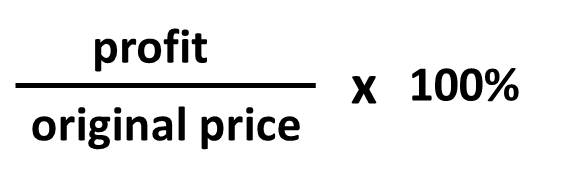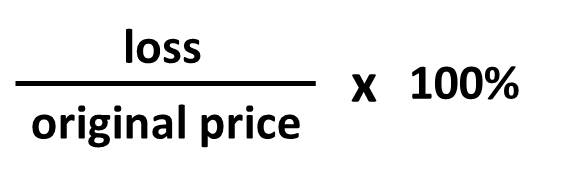## Example 1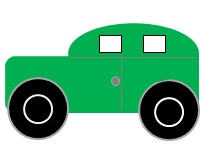John bought a car for ?12,500 five years a go. He sells the car to Mary for ?9,000.

Loss made: 12500 - 9000 = ?3,500

To find the loss made in percentage we would use the loss formula.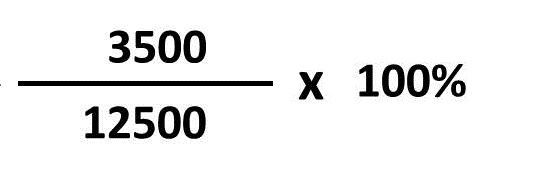## Example2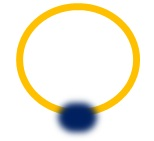Sara sold her saphire ring to a jewellery shop for ?1,450. She bought the ring 6 years ago for ?850.

Profit made: 1450 - 850 = ?600

To find the profit made in percentage we would use the profit formula.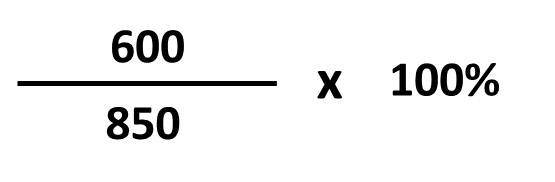# Fractions to Percentages

Example 1: 5/10 = ?

1. Fraction to Decimal: 5/10 = 0.5

2. Mulitply by 100: 0.5 x 100 = 50

Example 2: 1/4 = ?

1. Fraction to Decimal: 1/4 = 0.25

2. Mulitply by 100: 0.25 x 100 = 25

# Percentages to Fractions

Example 1: 75% = ?

1. Divide 75 by 100: 75/100

2. Simplify 75/100 = 3/4

Example 2: 45% = ?

1. Divide 45 by 100: 45/100

2. Simplify 45/100 = 9/20